Modules

Parallel Processing

Functions

template<... >
void convolveFFT (...)
Convolve an array with a kernel by means of the Fourier transform. More...

template<... >
void convolveFFTComplex (...)
Convolve a complex-valued array by means of the Fourier transform. More...

template<... >
void convolveFFTComplexMany (...)
Convolve a complex-valued array with a sequence of kernels by means of the Fourier transform. More...

template<... >
void convolveFFTMany (...)
Convolve a real-valued array with a sequence of kernels by means of the Fourier transform. More...

template<... >
void convolveImage (...)
Convolve an image with the given kernel(s). More...

template<... >
Deprecated name of 2-dimensional normalized convolution, i.e. convolution with a mask image. More...

template<... >
void convolveMultiArrayOneDimension (...)
Convolution along a single dimension of a multi-dimensional arrays. More...

template<... >
void correlateFFT (...)
Correlate an array with a kernel by means of the Fourier transform. More...

template<... >
void gaussianDivergenceMultiArray (...)
Calculate the divergence of a vector field using Gaussian derivative filters. More...

template<... >
Calculate the gradient vector by means of a 1st derivatives of Gaussian filter. More...

template<... >
Calculate the gradient magnitude by means of a 1st derivatives of Gaussian filter. More...

template<... >
Calculate Gaussian gradient of a multi-dimensional arrays. More...

template<... >
void gaussianSharpening (...)
Perform sharpening function with gaussian filter. More...

template<... >
void gaussianSmoothing (...)
Perform isotropic Gaussian convolution. More...

template<... >
void gaussianSmoothMultiArray (...)
Isotropic Gaussian smoothing of a multi-dimensional arrays. More...

template<... >
void hessianMatrixOfGaussian (...)
Filter image with the 2nd derivatives of the Gaussian at the given scale to get the Hessian matrix. More...

template<... >
void hessianOfGaussianMultiArray (...)
Calculate Hessian matrix of a N-dimensional arrays using Gaussian derivative filters. More...

template<... >
void laplacianOfGaussian (...)
Filter image with the Laplacian of Gaussian operator at the given scale. More...

template<... >
void laplacianOfGaussianMultiArray (...)
Calculate Laplacian of a N-dimensional arrays using Gaussian derivative filters. More...

template<... >
void normalizedConvolveImage (...)
Performs a 2-dimensional normalized convolution, i.e. convolution with a mask image. More...

template<... >
void rieszTransformOfLOG (...)
Calculate Riesz transforms of the Laplacian of Gaussian. More...

template<... >
void separableConvolveMultiArray (...)
Separated convolution on multi-dimensional arrays. More...

template<... >
void simpleSharpening (...)
Perform simple sharpening function. More...

template<... >
void structureTensor (...)
Calculate the Structure Tensor for each pixel of and image, using Gaussian (derivative) filters. More...

template<... >
void structureTensorMultiArray (...)
Calculate the structure tensor of a multi-dimensional arrays. More...

template<... >
Calculate gradient of a multi-dimensional arrays using symmetric difference filters. More...

Detailed Description

The functions in this group implement separable convolutions (e.g. smoothing and sharpening, Gaussian derivatives) and related filters (like the gradient magnitude) on arbitrary-dimensional arrays. In addition, non-separable filters are supported for 2D images. All functions accept the vigra::MultiArrayView API (which can be wrapped around a wide variety of data structures) as well as a number of deprecated APIs such as Image Iterators.

Function Documentation

 void vigra::rieszTransformOfLOG ( ... )

Calculate Riesz transforms of the Laplacian of Gaussian.

The Riesz transforms of the Laplacian of Gaussian have the following transfer functions (defined in a polar coordinate representation of the frequency domain):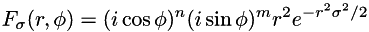where n = xorder and m = yorder determine th e order of the transform, and sigma > 0 is the scale of the Laplacian of Gaussian. This function computes a good spatial domain approximation of these transforms for xorder + yorder <= 2. The filter responses may be used to calculate the monogenic signal or the boundary tensor.

Declarations:

pass 2D array views:

namespace vigra {
template <class T1, class S1,
class T2, class S2>
void
rieszTransformOfLOG(MultiArrayView<2, T1, S1> const & src,
MultiArrayView<2, T2, S2> dest,
double scale, unsigned int xorder, unsigned int yorder);
}

show deprecated declarations

Usage:

#include <vigra/boundarytensor.hxx>
Namespace: vigra

MultiArrayView<2, double> impulse(17,17), res(17, 17);
impulse(8,8) = 1.0;
// calculate the impulse response of the first order Riesz transform in x-direction
rieszTransformOfLOG(impulse, res, 2.0, 1, 0);
 void vigra::convolveImage ( ... )

Convolve an image with the given kernel(s).

If you pass vigra::Kernel2D to this function, it will perform an explicit 2-dimensional convolution. If you pass a single vigra::Kernel1D, it performs a separable convolution, i.e. it concatenates two 1D convolutions (along the x-axis and along the y-axis) with the same kernel via internal calls to separableConvolveX() and separableConvolveY(). If two 1D kernels are specified, separable convolution uses different kernels for the x- and y-axis.

All border treatment modes are supported.

The input pixel type T1 must be a linear space over the kernel's value_type T, i.e. addition of source values, multiplication with kernel values, and NumericTraits must be defined. The kernel's value_type must be an algebraic field, i.e. the arithmetic operations (+, -, *, /) and NumericTraits must be defined. Typically, you will use double for the kernel type.

Declarations:

pass 2D array views:

namespace vigra {
// use the same 1D kernel for all axes
template <class T1, class S1,
class T2, class S2,
class T>
void
convolveImage(MultiArrayView<2, T1, S1> const & src,
MultiArrayView<2, T2, S2> dest,
Kernel1D<T> const & k);
// use a different kernel for each axis
template <class T1, class S1,
class T2, class S2,
class T>
void
convolveImage(MultiArrayView<2, T1, S1> const & src,
MultiArrayView<2, T2, S2> dest,
Kernel1D<T> const & kx, Kernel1D<T> const & ky);
// use a non-separable 2D kernel
template <class T1, class S1,
class T2, class S2,
class T3>
void
convolveImage(MultiArrayView<2, T1, S1> const & src,
MultiArrayView<2, T2, S2> dest,
Kernel2D<T3> const & kernel);
}

show deprecated declarations

Usage:

#include <vigra/convolution.hxx>
Namespace: vigra

MultiArray<2, float> src(w,h), dest1(w,h), dest2(w,h);
...
// create horizontal sobel filter (symmetric difference in x-direction, smoothing in y direction)
Kernel1D<double> kx, ky;
kx.initSymmetricDifference();
ky.initBinomial(1);
// calls separable convolution with the two 1D kernels
convolveImage(src, dest1, kx, ky);
// create a 3x3 Laplacian filter
Kernel2D<double> laplace;
laplace.initExplicitly(Diff2D(-1,-1), Diff2D(1,1)) =
0.375, 0.25, 0.375,
0.25, -2.5, 0.25,
0.375, 0.25, 0.375;
// calls 2D convolution
convolveImage(src, dest2, laplace);

show deprecated examples

Preconditions:

The image must be larger than the kernel radius.

• For 1D kernels, w > std::max(xkernel.right(), -xkernel.keft()) and h > std::max(ykernel.right(), -ykernel.left()) are required.
• For 2D kernels, w > std::max(kernel.lowerRight().x, -kernel.upperLeft().x) and h > std::max(kernel.lowerRight().y, -kernel.upperLeft().y) are required.

If BORDER_TREATMENT_CLIP is requested: the sum of kernel elements must be != 0.

Examples:
smooth_convolve.cxx.
 void vigra::simpleSharpening ( ... )

Perform simple sharpening function.

This function uses convolveImage() with the following 3x3 filter:

-sharpening_factor/16.0, -sharpening_factor/8.0, -sharpening_factor/16.0,
-sharpening_factor/8.0, 1.0+sharpening_factor*0.75, -sharpening_factor/8.0,
-sharpening_factor/16.0, -sharpening_factor/8.0, -sharpening_factor/16.0;

and uses BORDER_TREATMENT_REFLECT as border treatment mode.

Preconditions:

1. sharpening_factor >= 0
2. scale >= 0

Declarations:

pass 2D array views:

namespace vigra {
template <class T1, class S1,
class T2, class S2>
void
simpleSharpening(MultiArrayView<2, T1, S1> const & src,
MultiArrayView<2, T2, S2> dest,
double sharpening_factor);
}

show deprecated declarations

Usage:

#include <vigra/convolution.hxx>
Namespace: vigra

MultiArray<2, float> src(w,h), dest(w,h);
...
// sharpening with sharpening_factor = 0.1
vigra::simpleSharpening(src, dest, 0.1);

show deprecated examples

 void vigra::gaussianSharpening ( ... )

Perform sharpening function with gaussian filter.

This function uses gaussianSmoothing() at the given scale to create a temporary image 'smooth' and than blends the original and smoothed image according to the formula

dest = (1 + sharpening_factor)*src - sharpening_factor*smooth

Preconditions:

1. sharpening_factor >= 0
2. scale >= 0

Declarations:

pass 2D array views:

namespace vigra {
template <class T1, class S1,
class T2, class S2>
void
gaussianSharpening(MultiArrayView<2, T1, S1> const & src,
MultiArrayView<2, T2, S2> dest,
double sharpening_factor,
double scale);
}

show deprecated declarations

Usage:

#include <vigra/convolution.hxx>
Namespace: vigra

MultiArray<2, float> src(w,h), dest(w,h);
...
// sharpening with sharpening_factor = 3.0
// smoothing with scale = 0.5
gaussianSharpening(src, dest, 3.0, 0.5);

show deprecated examples

 void vigra::gaussianSmoothing ( ... )

Perform isotropic Gaussian convolution.

This function is a shorthand for the concatenation of a call to separableConvolveX() and separableConvolveY() with a Gaussian kernel of the given scale. If two scales are provided, smoothing in x and y direction will have different strength. The function uses BORDER_TREATMENT_REFLECT.

Function gaussianSmoothMultiArray() performs the same filter operation on arbitrary dimensional arrays.

Declarations:

pass 2D array views:

namespace vigra {
template <class T1, class S1,
class T2, class S2>
void
gaussianSmoothing(MultiArrayView<2, T1, S1> const & src,
MultiArrayView<2, T2, S2> dest,
double scale_x, double scale_y = scale_x);
}

show deprecated declarations

Usage:

#include <vigra/convolution.hxx>
Namespace: vigra

MultiArray<2, float> src(w,h), dest(w,h);
...
// smooth with scale = 3.0
gaussianSmoothing(src, dest, 3.0);

show deprecated examples

Examples:
smooth.cxx.
 void vigra::gaussianGradient ( ... )

Calculate the gradient vector by means of a 1st derivatives of Gaussian filter.

This function is a shorthand for the concatenation of a call to separableConvolveX() and separableConvolveY() with the appropriate kernels at the given scale. Note that this function can either produce two separate result images for the x- and y-components of the gradient, or write into a vector valued image (with at least two components).

Function gaussianGradientMultiArray() performs the same filter operation on arbitrary dimensional arrays.

Declarations:

pass 2D array views:

namespace vigra {
// write x and y component of the gradient into separate images
template <class T1, class S1,
class T2X, class S2X,
class T2Y, class S2Y>
void
gaussianGradient(MultiArrayView<2, T1, S1> const & src,
MultiArrayView<2, T2X, S2X> destx,
MultiArrayView<2, T2Y, S2Y> desty,
double scale);
// write x and y component of the gradient into a vector-valued image
template <class T1, class S1,
class T2, class S2>
void
gaussianGradient(MultiArrayView<2, T1, S1> const & src,
MultiArrayView<2, TinyVector<T2, 2>, S2> dest,
double scale);
}

show deprecated declarations

Usage:

#include <vigra/convolution.hxx>
Namespace: vigra

...
// calculate gradient vector at scale = 3.0
// likewise, but use a vector image to store the gradient
MultiArray<2, TinyVector<float, 2> > dest(w,h);

show deprecated examples

 void vigra::gaussianGradientMagnitude ( ... )

Calculate the gradient magnitude by means of a 1st derivatives of Gaussian filter.

This function calls gaussianGradient() and returns the pixel-wise magnitude of the resulting gradient vectors. If the original image has multiple bands, the squared gradient magnitude is computed for each band separately, and the return value is the square root of the sum of these squared magnitudes.

Anisotropic data should be provided with appropriate vigra::ConvolutionOptions to adjust the filter sizes for the resolution of each axis. Otherwise, the parameter opt is optional unless the parameter sigma is omitted.

If you pass vigra::BlockwiseConvolutionOptions instead, the algorithm will be executed in parallel on data blocks of a certain size. The block size can be customized via BlockwiseConvolutionOptions::blockShape(), but the defaults usually work reasonably. By default, the number of threads equals the capabilities of your hardware, but you can change this via BlockwiseConvolutionOptions::numThreads().

Declarations:

use arbitrary-dimensional arrays:

namespace vigra {
// pass filter scale explicitly
template <unsigned int N, class T1, class S1,
class T2, class S2>
void
gaussianGradientMagnitude(MultiArrayView<N, T1, S1> const & src,
MultiArrayView<N, T2, S2> dest,
double sigma,
ConvolutionOptions<N> opt = ConvolutionOptions<N>());
// likewise, but sum the contributions of each band
template <unsigned int N, class MT, class S1,
class T2, class S2>
void
gaussianGradientMagnitude(MultiArrayView<N+1, Multiband<MT>, S1> const & src,
MultiArrayView<N, T2, S2> dest,
double sigma,
ConvolutionOptions<N> opt = ConvolutionOptions<N>());
// pass filter scale(s) in option object
template <unsigned int N, class T1, class S1,
class T2, class S2>
void
gaussianGradientMagnitude(MultiArrayView<N, T1, S1> const & src,
MultiArrayView<N, T2, S2> dest,
ConvolutionOptions<N> const & opt);
// likewise, but execute algorithm in parallel
template <unsigned int N, class T1, class S1,
class T2, class S2>
void
gaussianGradientMagnitude(MultiArrayView<N, T1, S1> const & src,
MultiArrayView<N, T2, S2> dest,
BlockwiseConvolutionOptions<N> const & opt);
// pass filter scale(s) in option object and sum the contributions of each band
template <unsigned int N, class MT, class S1,
class T2, class S2>
void
gaussianGradientMagnitude(MultiArrayView<N+1, Multiband<MT>, S1> const & src,
MultiArrayView<N, T2, S2> dest,
ConvolutionOptions<N> const & opt);
}

Here, the input element types T1 and MT can be arbitrary scalar types, and T1 may also be TinyVector or RGBValue. The output element type T2 should be the corresponding norm type (see NormTraits). In the Multiband<MT>-version, the input array's right-most dimension is interpreted as a channel axis, therefore it must have one dimension more than the output array.

show deprecated declarations

Usage:

#include <vigra/multi_convolution.hxx> (sequential version)
#include <vigra/multi_blockwise.hxx> (parallel version)
#include <vigra/convolution.hxx> (deprecated API version)
Namespace: vigra

// example 1
{
// use a 3-dimensional float array
MultiArray<3, float> volume(Shape3(w, h, d)), grad(volume.shape());
...
// calculate gradient magnitude at scale = 3.0
}
// example 2
{
// use a 2-dimensional RGB array
MultiArray<2, RGBValue<float> > rgb(Shape2(w, h));
...
// calculate the color gradient magnitude at scale = 3.0
}
// example 3
{
// use a 3-dimensional array whose right-most axis is interpreted as
// a multi-spectral axis with arbitrary many channels
MultiArray<3, Multiband<float> > spectral(Shape3(w, h, channelCount));
MultiArray<2, float> grad(Shape2(w, h));
...
// calculate the multi-channel gradient magnitude at scale = 3.0
// (note that the template parameter N (number of spatial dimensions)
// must be provided explicitly as gaussianGradientMagnitude<2>(...) )
MultiArrayView<3, Multiband<float> > view(spectral);
}

show deprecated examples

Examples:
graph_agglomerative_clustering.cxx, and watershed.cxx.
 void vigra::laplacianOfGaussian ( ... )

Filter image with the Laplacian of Gaussian operator at the given scale.

This function calls separableConvolveX() and separableConvolveY() with the appropriate 2nd derivative of Gaussian kernels in x- and y-direction and then sums the results to get the Laplacian.

Function laplacianOfGaussianMultiArray() performs the same filter operation on arbitrary dimensional arrays.

Declarations:

pass 2D array views:

namespace vigra {
template <class T1, class S1,
class T2, class S2>
void
laplacianOfGaussian(MultiArrayView<2, T1, S1> const & src,
MultiArrayView<2, T2, S2> dest,
double scale);
}

show deprecated declarations

Usage:

#include <vigra/convolution.hxx>
Namespace: vigra

MultiArray<2, float> src(w,h), dest(w,h);
...
// calculate Laplacian of Gaussian at scale = 3.0
laplacianOfGaussian(src, dest, 3.0);

show deprecated examples

 void vigra::hessianMatrixOfGaussian ( ... )

Filter image with the 2nd derivatives of the Gaussian at the given scale to get the Hessian matrix.

The Hessian matrix is a symmetric matrix defined as: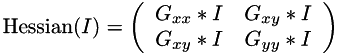where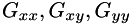denote 2nd derivatives of Gaussians at the given scale, and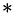is the convolution symbol. This function calls separableConvolveX() and separableConvolveY() with the appropriate 2nd derivative of Gaussian kernels and puts the results in the three destination images. The first destination image will contain the second derivative in x-direction, the second one the mixed derivative, and the third one holds the derivative in y-direction.

Function hessianOfGaussianMultiArray() performs the same filter operation on arbitrary dimensional arrays.

Declarations:

pass 2D array views:

namespace vigra {
template <class T1, class S1,
class T2, class S2>
void
hessianMatrixOfGaussian(MultiArrayView<2, T1, S1> const & src,
MultiArrayView<2, TinyVector<T2, 3>, S2> dest,
double scale);
}

show deprecated declarations

Usage:

#include <vigra/convolution.hxx>
Namespace: vigra

MultiArray<2, float> src(w,h);
MultiArray<2, TinyVector<float, 3> > hessian(w,h); // will hold the three components of the Hessian
...
// calculate Hessian of Gaussian at scale = 3.0, use a 3-band output image
hessianMatrixOfGaussian(src, hessian, 3.0);

show deprecated examples

 void vigra::structureTensor ( ... )

Calculate the Structure Tensor for each pixel of and image, using Gaussian (derivative) filters.

The Structure Tensor is is a smoothed version of the Euclidean product of the gradient vector with itself. I.e. it's a symmetric matrix defined as: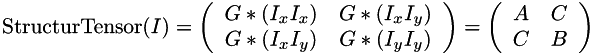where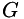denotes Gaussian smoothing at the outer scale,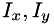are the gradient components taken at the inner scale,is the convolution symbol, and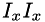etc. are pixelwise products of the 1st derivative images. This function calls separableConvolveX() and separableConvolveY() with the appropriate Gaussian kernels and puts the results in the three separate destination images (where the first one will contain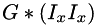, the second one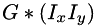, and the third one holds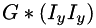), or into a single 3-band image (where the bands hold the result in the same order as above). The latter form is also applicable when the source image is a multi-band image (e.g. RGB). In this case, tensors are first computed for each band separately, and then summed up to get a single result tensor.

Function structureTensorMultiArray() performs the same filter operation on arbitrary dimensional arrays.

Declarations:

pass 2D array views:

namespace vigra {
// create three separate destination images
template <class T, class S,
class TX, class SX,
class TXY, class SXY,
class TY, class SY>
void
structureTensor(MultiArrayView<2, S, T> const & src,
MultiArrayView<2, TX, SX> destx,
MultiArrayView<2, TXY, SXY> destxy,
MultiArrayView<2, TY, SY> desty,
double inner_scale, double outer_scale);
// create a single 3-band destination image
template <class T1, class S1,
class T2, class S2>
void
structureTensor(MultiArrayView<2, T1, S1> const & src,
MultiArrayView<2, TinyVector<T2, 3>, S2> dest,
double inner_scale, double outer_scale);
}

show deprecated declarations

Usage:

#include <vigra/convolution.hxx>
Namespace: vigra

MultiArray<2, flost> src(w,h),
stxx(w,h), stxy(w,h), styy(w,h); // use a separate image for each component
...
// calculate Structure Tensor at inner scale = 1.0 and outer scale = 3.0
structureTensor(src, stxx, stxy, styy, 1.0, 3.0);
// likwise with a single 3-band destination image
MultiArray<2, TinyVector<float, 3> > st(w,h);
structureTensor(src, st, 1.0, 3.0);

show deprecated examples

 void vigra::separableConvolveMultiArray ( ... )

Separated convolution on multi-dimensional arrays.

This function computes a separated convolution on all dimensions of the given multi-dimensional array. Both source and destination arrays are represented by iterators, shape objects and accessors. The destination array is required to already have the correct size.

There are two variants of this functions: one takes a single kernel of type vigra::Kernel1D which is then applied to all dimensions, whereas the other requires an iterator referencing a sequence of vigra::Kernel1D objects, one for every dimension of the data. Then the first kernel in this sequence is applied to the innermost dimension (e.g. the x-axis of an image), while the last is applied to the outermost dimension (e.g. the z-axis in a 3D image).

This function may work in-place, which means that source.data() == dest.data() is allowed. A full-sized internal array is only allocated if working on the destination array directly would cause round-off errors (i.e. if typeid(typename NumericTraits<T2>::RealPromote) != typeid(T2)).

If start and stop have non-default values, they must represent a valid subarray of the input array. The convolution is then restricted to that subarray, and it is assumed that the output array only refers to the subarray (i.e. dest.shape() == stop - start). Negative ROI boundaries are interpreted relative to the end of the respective dimension (i.e. if(stop[k] < 0) stop[k] += source.shape(k);).

Declarations:

pass arbitrary-dimensional array views:

namespace vigra {
// apply each kernel from the sequence 'kernels' in turn
template <unsigned int N, class T1, class S1,
class T2, class S2,
class KernelIterator>
void
separableConvolveMultiArray(MultiArrayView<N, T1, S1> const & source,
MultiArrayView<N, T2, S2> dest,
KernelIterator kernels,
typename MultiArrayShape<N>::type start = typename MultiArrayShape<N>::type(),
typename MultiArrayShape<N>::type stop = typename MultiArrayShape<N>::type());
// apply the same kernel to all dimensions
template <unsigned int N, class T1, class S1,
class T2, class S2,
class T>
void
separableConvolveMultiArray(MultiArrayView<N, T1, S1> const & source,
MultiArrayView<N, T2, S2> dest,
Kernel1D<T> const & kernel,
typename MultiArrayShape<N>::type const & start = typename MultiArrayShape<N>::type(),
typename MultiArrayShape<N>::type const & stop = typename MultiArrayShape<N>::type());
}

show deprecated declarations

Usage:

#include <vigra/multi_convolution.hxx>
Namespace: vigra

Shape3 shape(width, height, depth);
MultiArray<3, unsigned char> source(shape);
MultiArray<3, float> dest(shape);
...
Kernel1D<float> gauss;
gauss.initGaussian(sigma);
// smooth all dimensions with the same kernel
separableConvolveMultiArray(source, dest, gauss);
// create 3 Gauss kernels, one for each dimension, but smooth the z-axis less
ArrayVector<Kernel1D<float> > kernels(3, gauss);
kernels.initGaussian(sigma / 2.0);
// perform Gaussian smoothing on all dimensions
separableConvolveMultiArray(source, dest, kernels.begin());
// create output array for a ROI
MultiArray<3, float> destROI(shape - Shape3(10,10,10));
// only smooth the given ROI (ignore 5 pixels on all sides of the array)
separableConvolveMultiArray(source, destROI, gauss, Shape3(5,5,5), Shape3(-5,-5,-5));

show deprecated examples

vigra::Kernel1D, convolveLine()
 void vigra::convolveMultiArrayOneDimension ( ... )

Convolution along a single dimension of a multi-dimensional arrays.

This function computes a convolution along one dimension (specified by the parameter dim of the given multi-dimensional array with the given kernel. The destination array must already have the correct size.

If start and stop have non-default values, they must represent a valid subarray of the input array. The convolution is then restricted to that subarray, and it is assumed that the output array only refers to the subarray (i.e. dest.shape() == stop - start). Negative ROI boundaries are interpreted relative to the end of the respective dimension (i.e. if(stop[k] < 0) stop[k] += source.shape(k);).

This function may work in-place, which means that source.data() == dest.data() is allowed.

Declarations:

pass arbitrary-dimensional array views:

namespace vigra {
template <unsigned int N, class T1, class S1,
class T2, class S2,
class T>
void
convolveMultiArrayOneDimension(MultiArrayView<N, T1, S1> const & source,
MultiArrayView<N, T2, S2> dest,
unsigned int dim,
Kernel1D<T> const & kernel,
typename MultiArrayShape<N>::type start = typename MultiArrayShape<N>::type(),
typename MultiArrayShape<N>::type stop = typename MultiArrayShape<N>::type());
}

show deprecated declarations

Usage:

#include <vigra/multi_convolution.hxx>
Namespace: vigra

Shape3 shape(width, height, depth);
MultiArray<3, unsigned char> source(shape);
MultiArray<3, float> dest(shape);
...
Kernel1D<float> gauss;
gauss.initGaussian(sigma);
// perform Gaussian smoothing along dimension 1 (height)
convolveMultiArrayOneDimension(source, dest, 1, gauss);
separableConvolveMultiArray()
 void vigra::gaussianSmoothMultiArray ( ... )

Isotropic Gaussian smoothing of a multi-dimensional arrays.

This function computes an isotropic convolution of the given N-dimensional array with a Gaussian filter at the given standard deviation sigma. Both source and destination arrays are represented by iterators, shape objects and accessors. The destination array is required to already have the correct size. This function may work in-place, which means that source.data() == dest.data() is allowed. It is implemented by a call to separableConvolveMultiArray() with the appropriate kernel.

Anisotropic data should be provided with appropriate vigra::ConvolutionOptions to adjust the filter sizes for the resolution of each axis. Otherwise, the parameter opt is optional unless the parameter sigma is omitted.

If you pass vigra::BlockwiseConvolutionOptions instead, the algorithm will be executed in parallel on data blocks of a certain size. The block size can be customized via BlockwiseConvolutionOptions::blockShape(), but the defaults usually work reasonably. By default, the number of threads equals the capabilities of your hardware, but you can change this via BlockwiseConvolutionOptions::numThreads().

Declarations:

pass arbitrary-dimensional array views:

namespace vigra {
// pass filter scale explicitly
template <unsigned int N, class T1, class S1,
class T2, class S2>
void
gaussianSmoothMultiArray(MultiArrayView<N, T1, S1> const & source,
MultiArrayView<N, T2, S2> dest,
double sigma,
ConvolutionOptions<N> opt = ConvolutionOptions<N>());
// pass filer scale(s) in the option object
template <unsigned int N, class T1, class S1,
class T2, class S2>
void
gaussianSmoothMultiArray(MultiArrayView<N, T1, S1> const & source,
MultiArrayView<N, T2, S2> dest,
ConvolutionOptions<N> opt);
// as above, but execute algorithm in parallel
template <unsigned int N, class T1, class S1,
class T2, class S2>
void
gaussianSmoothMultiArray(MultiArrayView<N, T1, S1> const & source,
MultiArrayView<N, T2, S2> dest,
BlockwiseConvolutionOptions<N> opt);
}

show deprecated declarations

Usage:

#include <vigra/multi_convolution.hxx> (sequential version)
#include <vigra/multi_blockwise.hxx> (parallel version)
Namespace: vigra

Shape3 shape(width, height, depth);
MultiArray<3, unsigned char> source(shape);
MultiArray<3, float> dest(shape);
...
// perform isotropic Gaussian smoothing at scale 'sigma'
gaussianSmoothMultiArray(source, dest, sigma);

Shape3 shape(width, height, depth);
MultiArray<3, unsigned char> source(shape);
MultiArray<3, float> dest(shape);
...
BlockwiseConvolutionOptions<3> opt;
opt.numThreads(4); // use 4 threads (uses hardware default if not given)
opt.innerScale(sigma);
// perform isotropic Gaussian smoothing at scale 'sigma' in parallel
gaussianSmoothMultiArray(source, dest, sigma, opt);

Usage with anisotropic data:

Shape3 shape(width, height, depth);
MultiArray<3, unsigned char> source(shape);
MultiArray<3, float> dest(shape);
TinyVector<float, 3> step_size;
TinyVector<float, 3> resolution_sigmas;
...
// perform anisotropic Gaussian smoothing at scale 'sigma'
gaussianSmoothMultiArray(source, dest, sigma,
ConvolutionOptions<3>().stepSize(step_size).resolutionStdDev(resolution_sigmas));
separableConvolveMultiArray()
 void vigra::gaussianGradientMultiArray ( ... )

Calculate Gaussian gradient of a multi-dimensional arrays.

This function computes the Gaussian gradient of the given N-dimensional array with a sequence of first-derivative-of-Gaussian filters at the given standard deviation sigma (differentiation is applied to each dimension in turn, starting with the innermost dimension). The destination array is required to have a vector valued pixel type with as many elements as the number of dimensions. This function is implemented by calls to separableConvolveMultiArray() with the appropriate kernels.

Anisotropic data should be provided with appropriate vigra::ConvolutionOptions to adjust the filter sizes for the resolution of each axis. Otherwise, the parameter opt is optional unless the parameter sigma is omitted.

If you pass vigra::BlockwiseConvolutionOptions instead, the algorithm will be executed in parallel on data blocks of a certain size. The block size can be customized via BlockwiseConvolutionOptions::blockShape(), but the defaults usually work reasonably. By default, the number of threads equals the capabilities of your hardware, but you can change this via BlockwiseConvolutionOptions::numThreads().

Declarations:

pass arbitrary-dimensional array views:

namespace vigra {
// pass filter scale explicitly
template <unsigned int N, class T1, class S1,
class T2, class S2>
void
gaussianGradientMultiArray(MultiArrayView<N, T1, S1> const & source,
MultiArrayView<N, TinyVector<T2, N>, S2> dest,
double sigma,
ConvolutionOptions<N> opt = ConvolutionOptions<N>());
// pass filter scale(s) in option object
template <unsigned int N, class T1, class S1,
class T2, class S2>
void
gaussianGradientMultiArray(MultiArrayView<N, T1, S1> const & source,
MultiArrayView<N, TinyVector<T2, N>, S2> dest,
ConvolutionOptions<N> opt);
// likewise, but execute algorithm in parallel
template <unsigned int N, class T1, class S1,
class T2, class S2>
void
gaussianGradientMultiArray(MultiArrayView<N, T1, S1> const & source,
MultiArrayView<N, TinyVector<T2, N>, S2> dest,
BlockwiseConvolutionOptions<N> opt);
}

show deprecated declarations

Usage:

#include <vigra/multi_convolution.hxx> (sequential version)
#include <vigra/multi_blockwise.hxx> (parallel version)
Namespace: vigra

Shape3 shape(width, height, depth);
MultiArray<3, unsigned char> source(shape);
MultiArray<3, TinyVector<float, 3> > dest(shape);
...
// compute Gaussian gradient at scale sigma

Usage with anisotropic data:

Shape3 shape(width, height, depth);
MultiArray<3, unsigned char> source(shape);
MultiArray<3, TinyVector<float, 3> > dest(shape);
TinyVector<float, 3> step_size;
TinyVector<float, 3> resolution_sigmas;
...
// compute Gaussian gradient at scale sigma
ConvolutionOptions<3>().stepSize(step_size).resolutionStdDev(resolution_sigmas));
separableConvolveMultiArray()
 void vigra::symmetricGradientMultiArray ( ... )

Calculate gradient of a multi-dimensional arrays using symmetric difference filters.

This function computes the gradient of the given N-dimensional array with a sequence of symmetric difference filters a (differentiation is applied to each dimension in turn, starting with the innermost dimension). The destination array is required to have a vector valued pixel type with as many elements as the number of dimensions. This function is implemented by calls to convolveMultiArrayOneDimension() with the symmetric difference kernel.

Anisotropic data should be provided with appropriate vigra::ConvolutionOptions to adjust the filter sizes for the resolution of each axis. Otherwise, the parameter opt is optional unless the parameter sigma is omitted.

If you pass vigra::BlockwiseConvolutionOptions instead, the algorithm will be executed in parallel on data blocks of a certain size. The block size can be customized via BlockwiseConvolutionOptions::blockShape(), but the defaults usually work reasonably. By default, the number of threads equals the capabilities of your hardware, but you can change this via BlockwiseConvolutionOptions::numThreads().

Declarations:

pass arbitrary-dimensional array views:

namespace vigra {
// execute algorithm sequentially
template <unsigned int N, class T1, class S1,
class T2, class S2>
void
symmetricGradientMultiArray(MultiArrayView<N, T1, S1> const & source,
MultiArrayView<N, TinyVector<T2, N>, S2> dest,
ConvolutionOptions<N> opt = ConvolutionOptions<N>());
// execute algorithm in parallel
template <unsigned int N, class T1, class S1,
class T2, class S2>
void
symmetricGradientMultiArray(MultiArrayView<N, T1, S1> const & source,
MultiArrayView<N, TinyVector<T2, N>, S2> dest,
BlockwiseConvolutionOptions<N> opt);
}

show deprecated declarations

Usage:

#include <vigra/multi_convolution.hxx> (sequential version)
#include <vigra/multi_blockwise.hxx> (parallel version)
Namespace: vigra

MultiArray<3, unsigned char>::size_type shape(width, height, depth);
MultiArray<3, unsigned char> source(shape);
MultiArray<3, TinyVector<float, 3> > dest(shape);
...

Usage with anisotropic data:

Shape3 shape(width, height, depth);
MultiArray<3, unsigned char> source(shape);
MultiArray<3, TinyVector<float, 3> > dest(shape);
TinyVector<float, 3> step_size;
...
ConvolutionOptions<3>().stepSize(step_size));
convolveMultiArrayOneDimension()
 void vigra::laplacianOfGaussianMultiArray ( ... )

Calculate Laplacian of a N-dimensional arrays using Gaussian derivative filters.

This function computes the Laplacian of the given N-dimensional array with a sequence of second-derivative-of-Gaussian filters at the given standard deviation sigma. Both source and destination arrays must have scalar value_type. This function is implemented by calls to separableConvolveMultiArray() with the appropriate kernels, followed by summation.

Anisotropic data should be provided with appropriate vigra::ConvolutionOptions to adjust the filter sizes for the resolution of each axis. Otherwise, the parameter opt is optional unless the parameter sigma is omitted.

If you pass vigra::BlockwiseConvolutionOptions instead, the algorithm will be executed in parallel on data blocks of a certain size. The block size can be customized via BlockwiseConvolutionOptions::blockShape(), but the defaults usually work reasonably. By default, the number of threads equals the capabilities of your hardware, but you can change this via BlockwiseConvolutionOptions::numThreads().

Declarations:

pass arbitrary-dimensional array views:

namespace vigra {
// pass scale explicitly
template <unsigned int N, class T1, class S1,
class T2, class S2>
void
laplacianOfGaussianMultiArray(MultiArrayView<N, T1, S1> const & source,
MultiArrayView<N, T2, S2> dest,
double sigma,
ConvolutionOptions<N> opt = ConvolutionOptions<N>());
// pass scale(s) in option object
template <unsigned int N, class T1, class S1,
class T2, class S2>
void
laplacianOfGaussianMultiArray(MultiArrayView<N, T1, S1> const & source,
MultiArrayView<N, T2, S2> dest,
ConvolutionOptions<N> opt );
// likewise, but execute algorithm in parallel
template <unsigned int N, class T1, class S1,
class T2, class S2>
void
laplacianOfGaussianMultiArray(MultiArrayView<N, T1, S1> const & source,
MultiArrayView<N, T2, S2> dest,
BlockwiseConvolutionOptions<N> opt );
}

show deprecated declarations

Usage:

#include <vigra/multi_convolution.hxx> (sequential version)
#include <vigra/multi_blockwise.hxx> (parallel version)
Namespace: vigra

Shape3 shape(width, height, depth);
MultiArray<3, float> source(shape);
MultiArray<3, float> laplacian(shape);
...
// compute Laplacian at scale sigma
laplacianOfGaussianMultiArray(source, laplacian, sigma);

Usage with anisotropic data:

MultiArray<3, float> source(shape);
MultiArray<3, float> laplacian(shape);
TinyVector<float, 3> step_size;
TinyVector<float, 3> resolution_sigmas;
...
// compute Laplacian at scale sigma
laplacianOfGaussianMultiArray(source, laplacian, sigma,
ConvolutionOptions<3>().stepSize(step_size).resolutionStdDev(resolution_sigmas));
separableConvolveMultiArray()
 void vigra::gaussianDivergenceMultiArray ( ... )

Calculate the divergence of a vector field using Gaussian derivative filters.

This function computes the divergence of the given N-dimensional vector field with a sequence of first-derivative-of-Gaussian filters at the given standard deviation sigma. The input vector field can either be given as a sequence of scalar array views (one for each vector field component), represented by an iterator range, or by a single vector array with the appropriate shape. This function is implemented by calls to separableConvolveMultiArray() with the suitable kernels, followed by summation.

Anisotropic data should be provided with appropriate vigra::ConvolutionOptions to adjust the filter sizes for the resolution of each axis. Otherwise, the parameter opt is optional unless the parameter sigma is omitted.

If you pass vigra::BlockwiseConvolutionOptions instead, the algorithm will be executed in parallel on data blocks of a certain size. The block size can be customized via BlockwiseConvolutionOptions::blockShape(), but the defaults usually work reasonably. By default, the number of threads equals the capabilities of your hardware, but you can change this via BlockwiseConvolutionOptions::numThreads().

Declarations:

pass arbitrary-dimensional array views:

namespace vigra {
// specify input vector field as a sequence of scalar arrays
template <class Iterator,
unsigned int N, class T, class S>
void
gaussianDivergenceMultiArray(Iterator vectorField, Iterator vectorFieldEnd,
MultiArrayView<N, T, S> divergence,
ConvolutionOptions<N> const & opt);
template <class Iterator,
unsigned int N, class T, class S>
void
gaussianDivergenceMultiArray(Iterator vectorField, Iterator vectorFieldEnd,
MultiArrayView<N, T, S> divergence,
double sigma,
ConvolutionOptions<N> opt = ConvolutionOptions<N>());
// pass input vector field as an array of vectors
template <unsigned int N, class T1, class S1,
class T2, class S2>
void
gaussianDivergenceMultiArray(MultiArrayView<N, TinyVector<T1, N>, S1> const & vectorField,
MultiArrayView<N, T2, S2> divergence,
ConvolutionOptions<N> const & opt);
template <unsigned int N, class T1, class S1,
class T2, class S2>
void
gaussianDivergenceMultiArray(MultiArrayView<N, TinyVector<T1, N>, S1> const & vectorField,
MultiArrayView<N, T2, S2> divergence,
double sigma,
ConvolutionOptions<N> opt = ConvolutionOptions<N>());
// pass input vector field as an array of vectors and
// execute algorithm in parallel
template <unsigned int N, class T1, class S1,
class T2, class S2>
void
gaussianDivergenceMultiArray(MultiArrayView<N, TinyVector<T1, N>, S1> const & vectorField,
MultiArrayView<N, T2, S2> divergence,
BlockwiseConvolutionOptions<N> const & opt);
}

Usage:

#include <vigra/multi_convolution.hxx> (sequential version)
#include <vigra/multi_blockwise.hxx> (parallel version)
Namespace: vigra

Shape3 shape(width, height, depth);
MultiArray<3, TinyVector<float, 3> > source(shape);
MultiArray<3, float> laplacian(shape);
...
// compute divergence at scale sigma
gaussianDivergenceMultiArray(source, laplacian, sigma);

Usage with anisotropic data:

MultiArray<3, TinyVector<float, 3> > source(shape);
MultiArray<3, float> laplacian(shape);
TinyVector<float, 3> step_size;
TinyVector<float, 3> resolution_sigmas;
...
// compute divergence at scale sigma
gaussianDivergenceMultiArray(source, laplacian, sigma,
ConvolutionOptions<3>().stepSize(step_size).resolutionStdDev(resolution_sigmas));
 void vigra::hessianOfGaussianMultiArray ( ... )

Calculate Hessian matrix of a N-dimensional arrays using Gaussian derivative filters.

This function computes the Hessian matrix the given scalar N-dimensional array with a sequence of second-derivative-of-Gaussian filters at the given standard deviation sigma. The destination array must have a vector valued element type with N*(N+1)/2 elements (it represents the upper triangular part of the symmetric Hessian matrix, flattened row-wise). This function is implemented by calls to separableConvolveMultiArray() with the appropriate kernels.

Anisotropic data should be provided with appropriate vigra::ConvolutionOptions to adjust the filter sizes for the resolution of each axis. Otherwise, the parameter opt is optional unless the parameter sigma is omitted.

If you pass vigra::BlockwiseConvolutionOptions instead, the algorithm will be executed in parallel on data blocks of a certain size. The block size can be customized via BlockwiseConvolutionOptions::blockShape(), but the defaults usually work reasonably. By default, the number of threads equals the capabilities of your hardware, but you can change this via BlockwiseConvolutionOptions::numThreads().

Declarations:

pass arbitrary-dimensional array views:

namespace vigra {
// pass scale explicitly
template <unsigned int N, class T1, class S1,
class T2, class S2>
void
hessianOfGaussianMultiArray(MultiArrayView<N, T1, S1> const & source,
MultiArrayView<N, TinyVector<T2, int(N*(N+1)/2)>, S2> dest,
double sigma,
ConvolutionOptions<N> opt = ConvolutionOptions<N>());
// pass scale(s) in option object
template <unsigned int N, class T1, class S1,
class T2, class S2>
void
hessianOfGaussianMultiArray(MultiArrayView<N, T1, S1> const & source,
MultiArrayView<N, TinyVector<T2, int(N*(N+1)/2)>, S2> dest,
ConvolutionOptions<N> opt);
// likewise, but execute algorithm in parallel
template <unsigned int N, class T1, class S1,
class T2, class S2>
void
hessianOfGaussianMultiArray(MultiArrayView<N, T1, S1> const & source,
MultiArrayView<N, TinyVector<T2, int(N*(N+1)/2)>, S2> dest,
BlockwiseConvolutionOptions<N> opt);
}

show deprecated declarations

Usage:

#include <vigra/multi_convolution.hxx> (sequential version)
#include <vigra/multi_blockwise.hxx> (parallel version)
Namespace: vigra

Shape3 shape(width, height, depth);
MultiArray<3, float> source(shape);
MultiArray<3, TinyVector<float, 6> > dest(shape);
...
// compute Hessian at scale sigma
hessianOfGaussianMultiArray(source, dest, sigma);

Usage with anisotropic data:

MultiArray<3, float> source(shape);
MultiArray<3, TinyVector<float, 6> > dest(shape);
TinyVector<float, 3> step_size;
TinyVector<float, 3> resolution_sigmas;
...
// compute Hessian at scale sigma
hessianOfGaussianMultiArray(source, dest, sigma,
ConvolutionOptions<3>().stepSize(step_size).resolutionStdDev(resolution_sigmas));
separableConvolveMultiArray(), vectorToTensorMultiArray()
 void vigra::structureTensorMultiArray ( ... )

Calculate the structure tensor of a multi-dimensional arrays.

This function computes the gradient (outer product) tensor for each element of the given N-dimensional array with first-derivative-of-Gaussian filters at the given innerScale, followed by Gaussian smoothing at outerScale. The destination array must have a vector valued pixel type with N*(N+1)/2 elements (it represents the upper triangular part of the symmetric structure tensor matrix, flattened row-wise). If the source array is also vector valued, the resulting structure tensor is the sum of the individual tensors for each channel. This function is implemented by calls to separableConvolveMultiArray() with the appropriate kernels.

Anisotropic data should be provided with appropriate vigra::ConvolutionOptions to adjust the filter sizes for the resolution of each axis. Otherwise, the parameter opt is optional unless the parameters innerScale and outerScale are both omitted.

If you pass vigra::BlockwiseConvolutionOptions instead, the algorithm will be executed in parallel on data blocks of a certain size. The block size can be customized via BlockwiseConvolutionOptions::blockShape(), but the defaults usually work reasonably. By default, the number of threads equals the capabilities of your hardware, but you can change this via BlockwiseConvolutionOptions::numThreads().

Declarations:

pass arbitrary-dimensional array views:

namespace vigra {
// pass scales explicitly
template <unsigned int N, class T1, class S1,
class T2, class S2>
void
structureTensorMultiArray(MultiArrayView<N, T1, S1> const & source,
MultiArrayView<N, TinyVector<T2, int(N*(N+1)/2)>, S2> dest,
double innerScale, double outerScale,
ConvolutionOptions<N> opt = ConvolutionOptions<N>());
// pass scales in option object
template <unsigned int N, class T1, class S1,
class T2, class S2>
void
structureTensorMultiArray(MultiArrayView<N, T1, S1> const & source,
MultiArrayView<N, TinyVector<T2, int(N*(N+1)/2)>, S2> dest,
ConvolutionOptions<N> opt );
// likewise, but execute algorithm in parallel
template <unsigned int N, class T1, class S1,
class T2, class S2>
void
structureTensorMultiArray(MultiArrayView<N, T1, S1> const & source,
MultiArrayView<N, TinyVector<T2, int(N*(N+1)/2)>, S2> dest,
BlockwiseConvolutionOptions<N> opt );
}

show deprecated declarations

Usage:

#include <vigra/multi_convolution.hxx> (sequential version)
#include <vigra/multi_blockwise.hxx> (parallel version)
Namespace: vigra

Shape3 shape(width, height, depth);
MultiArray<3, RGBValue<float> > source(shape);
MultiArray<3, TinyVector<float, 6> > dest(shape);
...
// compute structure tensor at scales innerScale and outerScale
structureTensorMultiArray(source, dest, innerScale, outerScale);

Usage with anisotropic data:

MultiArray<3, RGBValue<float> > source(shape);
MultiArray<3, TinyVector<float, 6> > dest(shape);
TinyVector<float, 3> step_size;
TinyVector<float, 3> resolution_sigmas;
...
// compute structure tensor at scales innerScale and outerScale
structureTensorMultiArray(source, dest, innerScale, outerScale,
ConvolutionOptions<3>().stepSize(step_size).resolutionStdDev(resolution_sigmas));
separableConvolveMultiArray(), vectorToTensorMultiArray()
 void vigra::convolveFFT ( ... )

Convolve an array with a kernel by means of the Fourier transform.

Thanks to the convolution theorem of Fourier theory, a convolution in the spatial domain is equivalent to a multiplication in the frequency domain. Thus, for certain kernels (especially large, non-separable ones), it is advantageous to perform the convolution by first transforming both array and kernel to the frequency domain, multiplying the frequency representations, and transforming the result back into the spatial domain. Some kernels have a much simpler definition in the frequency domain, so that they are readily computed there directly, avoiding Fourier transformation of those kernels.

The following functions implement various variants of FFT-based convolution:

convolveFFT
Convolve a real-valued input array with a kernel such that the result is also real-valued. That is, the kernel is either provided as a real-valued array in the spatial domain, or as a complex-valued array in the Fourier domain, using the half-space format of the R2C Fourier transform (see below).
convolveFFTMany
Like convolveFFT, but you may provide many kernels at once (using an iterator pair specifying the kernel sequence). This has the advantage that the forward transform of the input array needs to be executed only once.
convolveFFTComplex
Convolve a complex-valued input array with a complex-valued kernel, resulting in a complex-valued output array. An additional flag is used to specify whether the kernel is defined in the spatial or frequency domain.
convolveFFTComplexMany
Like convolveFFTComplex, but you may provide many kernels at once (using an iterator pair specifying the kernel sequence). This has the advantage that the forward transform of the input array needs to be executed only once.

The output arrays must have the same shape as the input arrays. In the "Many" variants of the convolution functions, the kernels must all have the same shape.

The origin of the kernel is always assumed to be in the center of the kernel array (precisely, at the point floor(kernel.shape() / 2.0), except when the half-space format is used, see below). The function moveDCToUpperLeft() will be called internally to align the kernel with the transformed input as appropriate.

If a real input is combined with a real kernel, the kernel is automatically assumed to be defined in the spatial domain. If a real input is combined with a complex kernel, the kernel is assumed to be defined in the Fourier domain in half-space format. If the input array is complex, a flag fourierDomainKernel determines where the kernel is defined.

When the kernel is defined in the spatial domain, the convolution functions will automatically pad (enlarge) the input array by at least the kernel radius in each direction. The newly added space is filled according to reflective boundary conditions in order to minimize border artifacts during convolution. It is thus ensured that convolution in the Fourier domain yields the same results as convolution in the spatial domain (e.g. when separableConvolveMultiArray() is called with the same kernel). A little further padding may be added to make sure that the padded array shape uses integers which have only small prime factors, because FFTW is then able to use the fastest possible algorithms. Any padding is automatically removed from the result arrays before the function returns.

When the kernel is defined in the frequency domain, it must be complex-valued, and its shape determines the shape of the Fourier representation (i.e. the input is padded according to the shape of the kernel). If we are going to perform a complex-valued convolution, the kernel must be defined for the entire frequency domain, and its shape directly determines the size of the FFT.

In contrast, a frequency domain kernel for a real-valued convolution must have symmetry properties that allow to drop half of the kernel coefficients, as in the R2C transform. That is, the kernel must have the half-space format, that is the shape returned by fftwCorrespondingShapeR2C(fourier_shape), where fourier_shape is the desired logical shape of the frequency representation (and thus the size of the padded input). The origin of the kernel must be at the point (0, floor(fourier_shape / 2.0), ..., floor(fourier_shape[N-1] / 2.0)) (i.e. as in a regular kernel except for the first dimension).

The Real type in the declarations can be double, float, and long double. Your program must always link against libfftw3. If you use float or long double arrays, you must additionally link against libfftw3f and libfftw3l respectively.

The Fourier transform functions internally create FFTW plans which control the algorithm details. The plans are created with the flag FFTW_ESTIMATE, i.e. optimal settings are guessed or read from saved "wisdom" files. If you need more control over planning, you can use the class FFTWConvolvePlan.

See also applyFourierFilter() for corresponding functionality on the basis of the old image iterator interface.

Declarations:

Real-valued convolution with kernel in the spatial domain:

namespace vigra {
template <unsigned int N, class Real, class C1, class C2, class C3>
void
convolveFFT(MultiArrayView<N, Real, C1> in,
MultiArrayView<N, Real, C2> kernel,
MultiArrayView<N, Real, C3> out);
}

Real-valued convolution with kernel in the Fourier domain (half-space format):

namespace vigra {
template <unsigned int N, class Real, class C1, class C2, class C3>
void
convolveFFT(MultiArrayView<N, Real, C1> in,
MultiArrayView<N, FFTWComplex<Real>, C2> kernel,
MultiArrayView<N, Real, C3> out);
}

Series of real-valued convolutions with kernels in the spatial or Fourier domain (the kernel and out sequences must have the same length):

namespace vigra {
template <unsigned int N, class Real, class C1,
class KernelIterator, class OutIterator>
void
convolveFFTMany(MultiArrayView<N, Real, C1> in,
KernelIterator kernels, KernelIterator kernelsEnd,
OutIterator outs);
}

Complex-valued convolution (parameter fourierDomainKernel determines if the kernel is defined in the spatial or Fourier domain):

namespace vigra {
template <unsigned int N, class Real, class C1, class C2, class C3>
void
convolveFFTComplex(MultiArrayView<N, FFTWComplex<Real>, C1> in,
MultiArrayView<N, FFTWComplex<Real>, C2> kernel,
MultiArrayView<N, FFTWComplex<Real>, C3> out,
bool fourierDomainKernel);
}

Series of complex-valued convolutions (parameter fourierDomainKernel determines if the kernels are defined in the spatial or Fourier domain, the kernel and out sequences must have the same length):

namespace vigra {
template <unsigned int N, class Real, class C1,
class KernelIterator, class OutIterator>
void
convolveFFTComplexMany(MultiArrayView<N, FFTWComplex<Real>, C1> in,
KernelIterator kernels, KernelIterator kernelsEnd,
OutIterator outs,
bool fourierDomainKernel);
}

Usage:

#include <vigra/multi_fft.hxx>
Namespace: vigra

// convolve real array with a Gaussian (sigma=1) defined in the spatial domain
// (implicitly uses padding by at least 4 pixels)
MultiArray<2, double> src(Shape2(w, h)), dest(Shape2(w,h));
MultiArray<2, double> spatial_kernel(Shape2(9, 9));
Gaussian<double> gauss(1.0);
for(int y=0; y<9; ++y)
for(int x=0; x<9; ++x)
spatial_kernel(x, y) = gauss(x-4.0)*gauss(y-4.0);
convolveFFT(src, spatial_kernel, dest);
// convolve real array with a Gaussian (sigma=1) defined in the Fourier domain
// (uses no padding, because the kernel size corresponds to the input size)
MultiArray<2, FFTWComplex<double> > fourier_kernel(fftwCorrespondingShapeR2C(src.shape()));
int y0 = h / 2;
for(int y=0; y<fourier_kernel.shape(1); ++y)
for(int x=0; x<fourier_kernel.shape(0); ++x)
fourier_kernel(x, y) = exp(-0.5*sq(x / double(w))) * exp(-0.5*sq((y-y0)/double(h)));
convolveFFT(src, fourier_kernel, dest);
 void vigra::convolveFFTComplex ( ... )

Convolve a complex-valued array by means of the Fourier transform.

See convolveFFT() for details.

 void vigra::convolveFFTMany ( ... )

Convolve a real-valued array with a sequence of kernels by means of the Fourier transform.

See convolveFFT() for details.

 void vigra::convolveFFTComplexMany ( ... )

Convolve a complex-valued array with a sequence of kernels by means of the Fourier transform.

See convolveFFT() for details.

 void vigra::correlateFFT ( ... )

Correlate an array with a kernel by means of the Fourier transform.

This function correlates a real-valued input array with a real-valued kernel such that the result is also real-valued. Thanks to the correlation theorem of Fourier theory, a correlation in the spatial domain is equivalent to a multiplication with the complex conjugate in the frequency domain. Thus, for certain kernels (especially large, non-separable ones), it is advantageous to perform the correlation by first transforming both array and kernel to the frequency domain, multiplying the frequency representations, and transforming the result back into the spatial domain.

The output arrays must have the same shape as the input arrays.

Declarations:

namespace vigra {
template <unsigned int N, class Real, class C1, class C2, class C3>
void
correlateFFT(MultiArrayView<N, Real, C1> in,
MultiArrayView<N, Real, C2> kernel,
MultiArrayView<N, Real, C3> out);
}

Usage:

#include <vigra/multi_fft.hxx>
Namespace: vigra

// correlate real array with a template to find best matches
// (implicitly uses padding by at least 4 pixels)
MultiArray<2, double> src(Shape2(w, h)), dest(Shape2(w, h));
MultiArray<2, double> template(Shape2(9, 9));
template = ...;
correlateFFT(src, template, dest);
 void vigra::normalizedConvolveImage ( ... )

Performs a 2-dimensional normalized convolution, i.e. convolution with a mask image.

This functions computes normalized convolution as defined in Knutsson, H. and Westin, C-F.: Normalized and differential convolution: Methods for Interpolation and Filtering of incomplete and uncertain data. Proc. of the IEEE Conf. on Computer Vision and Pattern Recognition, 1993, 515-523.

The mask image must be binary and encodes which pixels of the original image are valid. It is used as follows: Only pixel under the mask are used in the calculations. Whenever a part of the kernel lies outside the mask, it is ignored, and the kernel is renormalized to its original norm (analogous to the CLIP BorderTreatmentMode). Thus, a useful convolution result is computed whenever at least one valid pixel is within the current window Thus, destination pixels not under the mask still receive a value if they are near the mask. Therefore, this algorithm is useful as an interpolator of sparse input data. If you are only interested in the destination values under the mask, you can perform a subsequent copyImageIf().

The KernelIterator must point to the center of the kernel, and the kernel's size is given by its upper left (x and y of distance <= 0) and lower right (distance >= 0) corners. The image must always be larger than the kernel. At those positions where the kernel does not completely fit into the image, the specified BorderTreatmentMode is applied. Only BORDER_TREATMENT_CLIP and BORDER_TREATMENT_AVOID are currently supported.

The images's pixel type (SrcAccessor::value_type) must be a linear space over the kernel's value_type (KernelAccessor::value_type), i.e. addition of source values, multiplication with kernel values, and NumericTraits must be defined. The kernel's value_type must be an algebraic field, i.e. the arithmetic operations (+, -, *, /) and NumericTraits must be defined.

Declarations:

pass 2D array views:

namespace vigra {
template <class T1, class S1,
class T2, class S2,
class TM, class SM,
class T3>
void
normalizedConvolveImage(MultiArrayView<2, T1, S1> const & src,
MultiArrayView<2, TM, SM> const & mask,
MultiArrayView<2, T2, S2> dest,
Kernel2D<T3> const & kernel);
}

show deprecated declarations

Usage:

#include <vigra/stdconvolution.hxx>
Namespace: vigra

MultiArray<2, float> src(w,h), dest(w,h);
MultiArray<2, unsigned char> mask(w,h);
...
// define 3x3 binomial filter
vigra::Kernel2D<float> binom;
binom.initExplicitly(Diff2D(-1,-1), Diff2D(1,1)) = // upper left and lower right
0.0625, 0.125, 0.0625,
0.125, 0.25, 0.125,
0.0625, 0.125, 0.0625;
normalizedConvolveImage(src, mask, dest, binom);

show deprecated examples

Preconditions:

• The image must be longer than the kernel radius: w > std::max(kernel.lowerRight().x, -kernel.upperLeft().x) and h > std::max(kernel.lowerRight().y, -kernel.upperLeft().y).
• The sum of kernel elements must be != 0.
• border == BORDER_TREATMENT_CLIP || border == BORDER_TREATMENT_AVOID
 void vigra::convolveImageWithMask ( ... )

Deprecated name of 2-dimensional normalized convolution, i.e. convolution with a mask image.

See normalizedConvolveImage() for documentation.

Declarations:

pass 2D array views:

namespace vigra {
template <class SrcIterator, class SrcAccessor,